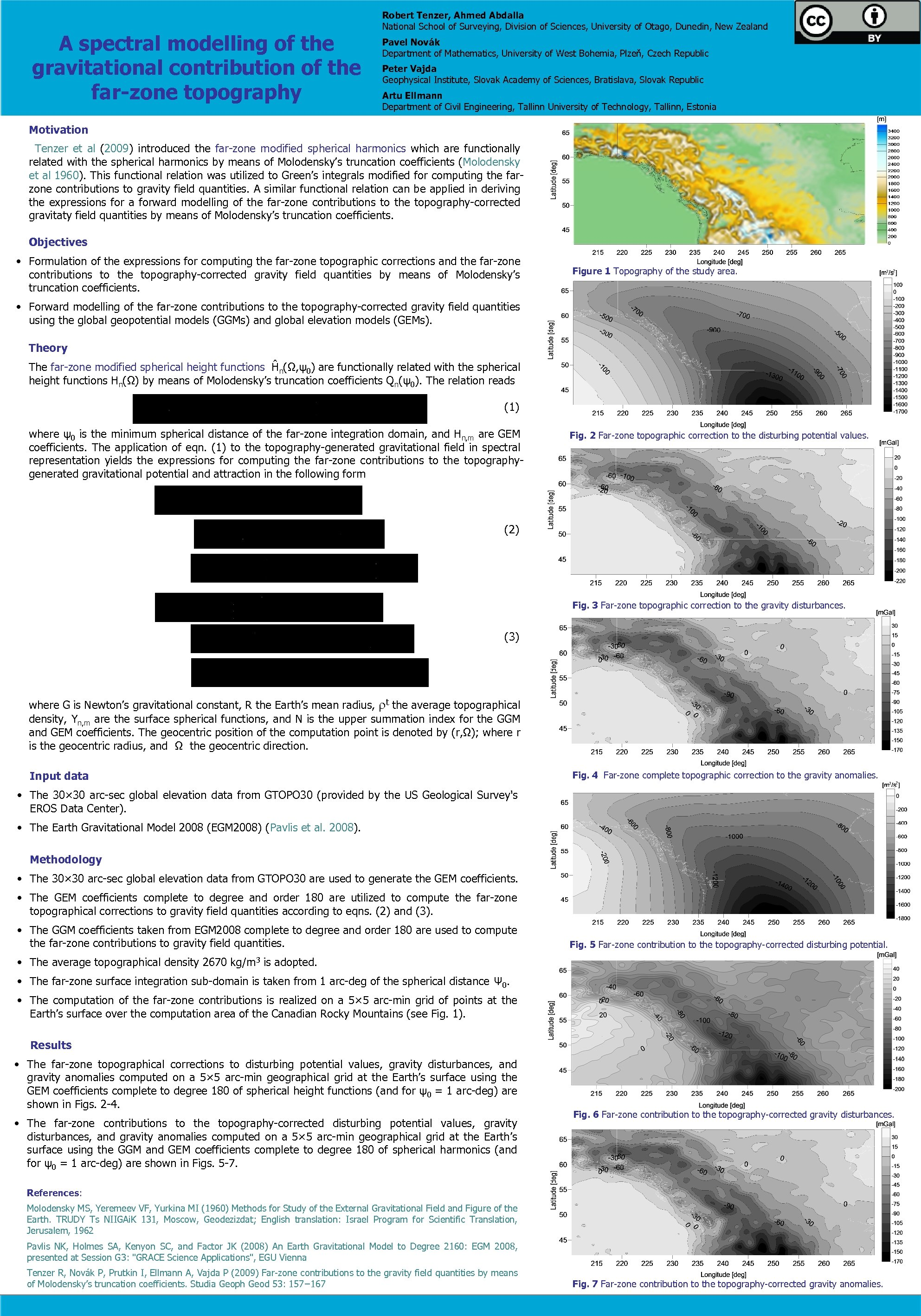Скачать презентацию A spectral modelling of the gravitational contribution of

23ead28a237bb54f5a9f5882d9432ee1.ppt

• Количество слайдов: 1A spectral modelling of the gravitational contribution of the far-zone topography Robert Tenzer, Ahmed Abdalla National School of Surveying, Division of Sciences, University of Otago, Dunedin, New Zealand Pavel Novák Department of Mathematics, University of West Bohemia, Plzeň, Czech Republic Peter Vajda Geophysical Institute, Slovak Academy of Sciences, Bratislava, Slovak Republic Artu Ellmann Department of Civil Engineering, Tallinn University of Technology, Tallinn, Estonia Motivation Tenzer et al (2009) introduced the far-zone modified spherical harmonics which are functionally related with the spherical harmonics by means of Molodensky’s truncation coefficients (Molodensky et al 1960). This functional relation was utilized to Green’s integrals modified for computing the farzone contributions to gravity field quantities. A similar functional relation can be applied in deriving the expressions for a forward modelling of the far-zone contributions to the topography-corrected gravitaty field quantities by means of Molodensky’s truncation coefficients. Objectives • Formulation of the expressions for computing the far-zone topographic corrections and the far-zone contributions to the topography-corrected gravity field quantities by means of Molodensky’s truncation coefficients. Figure 1 Topography of the study area. • Forward modelling of the far-zone contributions to the topography-corrected gravity field quantities using the global geopotential models (GGMs) and global elevation models (GEMs). Theory The far-zone modified spherical height functions Ĥn(Ω, ψ0) are functionally related with the spherical height functions Hn(Ω) by means of Molodensky’s truncation coefficients Qn(ψ0). The relation reads (1) where ψ0 is the minimum spherical distance of the far-zone integration domain, and Hn, m are GEM coefficients. The application of eqn. (1) to the topography-generated gravitational field in spectral representation yields the expressions for computing the far-zone contributions to the topographygenerated gravitational potential and attraction in the following form Fig. 2 Far-zone topographic correction to the disturbing potential values. (2) Fig. 3 Far-zone topographic correction to the gravity disturbances. (3) where G is Newton’s gravitational constant, R the Earth’s mean radius, t the average topographical density, Yn, m are the surface spherical functions, and N is the upper summation index for the GGM and GEM coefficients. The geocentric position of the computation point is denoted by (r, Ω); where r is the geocentric radius, and Ω the geocentric direction. Input data Fig. 4 Far-zone complete topographic correction to the gravity anomalies. • The 30× 30 arc-sec global elevation data from GTOPO 30 (provided by the US Geological Survey's EROS Data Center). • The Earth Gravitational Model 2008 (EGM 2008) (Pavlis et al. 2008). Methodology • The 30× 30 arc-sec global elevation data from GTOPO 30 are used to generate the GEM coefficients. • The GEM coefficients complete to degree and order 180 are utilized to compute the far-zone topographical corrections to gravity field quantities according to eqns. (2) and (3). • The GGM coefficients taken from EGM 2008 complete to degree and order 180 are used to compute the far-zone contributions to gravity field quantities. Fig. 5 Far-zone contribution to the topography-corrected disturbing potential. • The average topographical density 2670 kg/m 3 is adopted. • The far-zone surface integration sub-domain is taken from 1 arc-deg of the spherical distance Ψ 0. • The computation of the far-zone contributions is realized on a 5× 5 arc-min grid of points at the Earth’s surface over the computation area of the Canadian Rocky Mountains (see Fig. 1). Results • The far-zone topographical corrections to disturbing potential values, gravity disturbances, and gravity anomalies computed on a 5× 5 arc-min geographical grid at the Earth’s surface using the GEM coefficients complete to degree 180 of spherical height functions (and for ψ0 = 1 arc-deg) are shown in Figs. 2 -4. • The far-zone contributions to the topography-corrected disturbing potential values, gravity disturbances, and gravity anomalies computed on a 5× 5 arc-min geographical grid at the Earth’s surface using the GGM and GEM coefficients complete to degree 180 of spherical harmonics (and for ψ0 = 1 arc-deg) are shown in Figs. 5 -7. Fig. 6 Far-zone contribution to the topography-corrected gravity disturbances. References: Molodensky MS, Yeremeev VF, Yurkina MI (1960) Methods for Study of the External Gravitational Field and Figure of the Earth. TRUDY Ts NIIGAi. K 131, Moscow, Geodezizdat; English translation: Israel Program for Scientific Translation, Jerusalem, 1962 Pavlis NK, Holmes SA, Kenyon SC, and Factor JK (2008) An Earth Gravitational Model to Degree 2160: EGM 2008, presented at Session G 3: "GRACE Science Applications", EGU Vienna Tenzer R, Novák P, Prutkin I, Ellmann A, Vajda P (2009) Far-zone contributions to the gravity field quantities by means of Molodensky’s truncation coefficients. Studia Geoph Geod 53: 157− 167 Fig. 7 Far-zone contribution to the topography-corrected gravity anomalies.